### AME 301: Differential Equations, Vibrations and Control Homework 3

 B. GoodwineJ. Lucey Fall, 2003 Issued: September 10, 2003 Due: September 17, 2003 (US)18 September 2003 (UK)

1. (10 points) Show that the equivalent spring constant for the system illustrated in the following figure is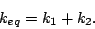Assume that the mass does not twist.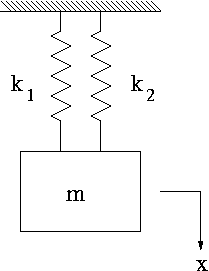Hint: both springs are stretched by the same amount.

2. (10 points) Show that the equivalent spring constant for the system illustrated in the following figure is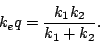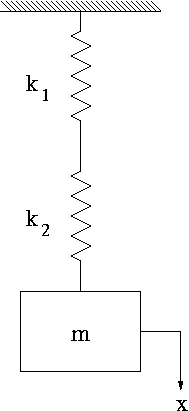Hint: both springs are subjected to the same force.

3. (10 points) Using your results from the previous two problems, determine the equivalent spring constant for the system in the following figure.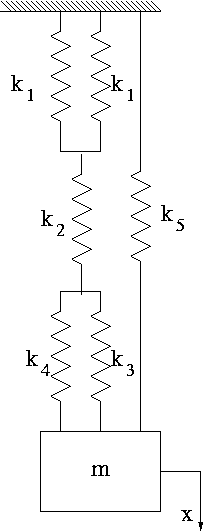4. (10 points) Consider the fixed-fixed beam system illustrated in the following figure.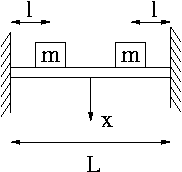Determine the equation of motion of the system with respect to the variable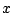. Assume that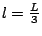and that the mass of the beam can be neglected.

Hint: First find the equivalent mass located directly over. You can assume that the deflection of the beam at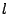is two-thirds of the deflection of the beam at the middle.

5. Consider the second order differential equation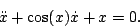1. (10 points) Is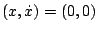an equilibrium point?
2. (10 points) Determine the best linear approximation to the equation aboutusing the Taylor series expansion approach.
3. (10 points) Determine the best linear approximation to the equation aboutusing the Jacobian approach.
6. (10 points) Consider the van der Pol equation, which is a model of an electric circuit that appeared in early radios with vacuum tubes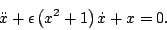Verify thatis an equilibrium point and determine the best linear approximation to the equation about that point.

7. Section 3.5:
1. (10 points) 3
2. (10 points) 5
3. (10 points) 11
4. (10 points) 12
5. (10 points) 18

Last updated: September 10, 2003.
B. Goodwine (goodwine@controls.ame.nd.edu)
J. Lucey (jlucey@nd.edu)